## Tamilnadu Samacheer Kalvi 6th Maths Solutions Term 3 Chapter 5 Information Processing Ex 5.2

Miscellaneous Practice problems

Question 1.
Find HCF of 188 and 230 by Euclid’s game.
Solution:
By Euclid’s game HCF (a, b) = HCF (a, a – b) if a > b.
Here HCF (188, 230) = HCF (230, – 188) because 230 > 188
= HCF (188, 42) = HCF (146, 42)
= HCF (104, 42) = HCF (62, 42)
= HCF (42, 20) = HCF (22, 20)
= HCF (20,2) = HCF (18, 2) = 2
∴ HCF (230, 188) = 2

Question 2.
Write the numbers from 1 to 50. From that find the following.
(i) The numbers which are neither divisible by 2 nor 7.
(ii) The prime numbers between 25 and 40.
(iii) All square number upto 50.
Solution :
(i) 9, 11, 13, 15, 17, 19, 23, 25, 27, 29, 31, 33, 37, 39, 41, 43, 45, 47
(ii) 29, 31, 37
(iii) 1, 4, 9, 16, 25, 36, 49Question 3.
Complete the following pattern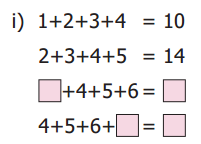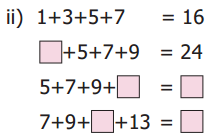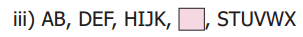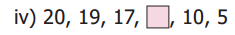Solution:
(i)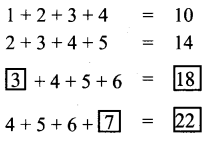(ii)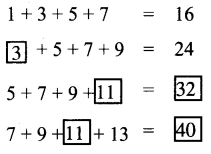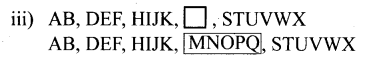Question 4.
Complete the table by using the following instructions.
A : It is the 6th term in the Fibonacci sequence.
B : The predecessor of 2.
C : LCM of 2 and 3.
D : HCF of 6 and 20.
E : The reciprocal of 1/5.
F : The opposite number of -7.
G : The first composite number.
H : Area of a square of side 3 cm.
I : The number of lines of symmetry of an equilateral triangle. After completing the table, what do you observe? Discuss.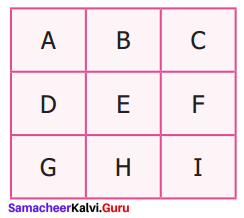Solution:
A : 6th term in Fibonacci sequence is 8.
B : Predecessor of 2 is 1.
C : LCM of 2 and 3 is 6.
D : HCF of 6 and 20 is 2.
E : Reciprocal of $$\frac{1}{5}$$ is 5.
F : Opposite number of – 7 is 7.
G : The first composite number is 4.
H : Area of square of side 3 cm is 3 × 3 = 9 cm2.
I : The number of lines of symmetry of an equilateral triangle is 3.
∴ The table becomes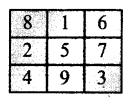From the table we observe that the numbers are from 1 to 9

Question 5.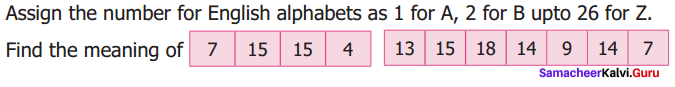Solution: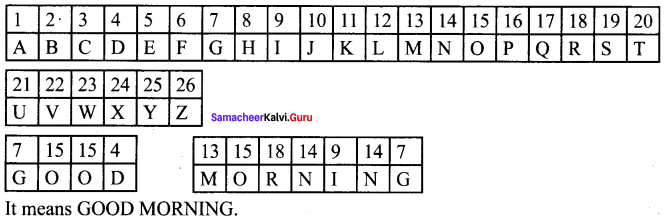Question 6.
Replace the letter by symbols as + for A, – for B, × for C and ÷ for D. Find the answer for the pattern 4B3C5A30D2 by doing the given operations.
Solution:
4

Question 7.
Observe the pattern and find the word by hiding the numbers
1H2O3W 4A5R6E 7Y809U?
Solution:
When hiding the numbers we get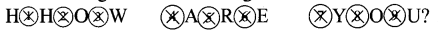HOW ARE YOU?Question 8.
Arranging from eldest to the youngest. What do you get ?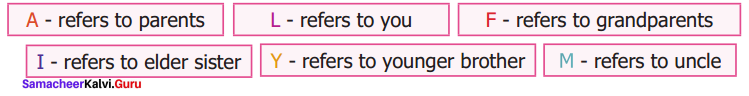Solution:
Arranging from eldest to the youngest we get
F – refers to grandparents
A – refers to parents
M – refers to uncle
I – refers to elder sister
L – refers to me
Y – refers to younger brother
So we get FAMILY

Challenge Problems

Question 9.
Prepare a daily time schedule for evening study at home.
Solution:
5.30 pm – arrival
5.30 pm – 6.30 pm – Tea, Tv programme
6.30 pm – 7.30 pm – Maths
7.30 pm – 8.30 pm – Supper, Tv news
8.30 pm – 9.00 pm – English
9.00 pm – 9.30 pm – Science
9.30 pm – 10.00 pm – Social science
10.00 pm – Going to bed.

Question 10.
Observe the geometrical pattern and answer the following questions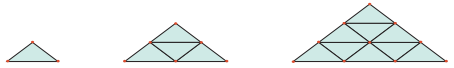i) Write down the number of sticks used in each of the iterative pattern.
ii) Draw the next figure in the pattern also find the total number sticks used in it.
Solution:
Number of sticks used in first pattern = 3
Number of sticks in second pattern = 9
Number of sticks in third pattern =18
ii) Next pattern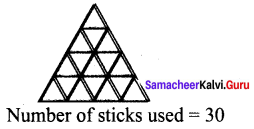Question 11.
Find HCF of 28y, 35, 42 by Euclid’s game.
Solution: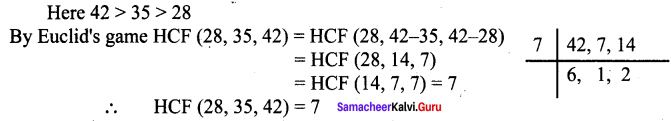Question 12.
Follow the given instructions to fill your name in the OMR sheet.
* The name should be written in capital letters from left to right.
* One alphabet is to be entered in each box.
* If any empty boxes are there at the end they should be left blank.
* Ball point pen is to be used for shading the bubbles for the corresponding alphabets.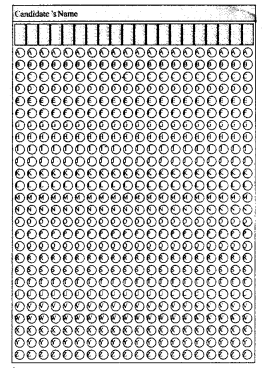Solution: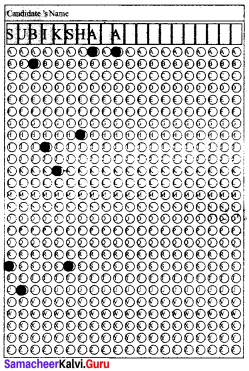Question 13.
Consider the Postal Index Number (PIN) written on the letters as follows: 604506; 604516; 604560; 604506; 604516; 604516; 604560; 604516; 604505; 604470; 604515; 604520; 604303; 604509; 604470. How the letters can be sorted as per Postal Index Numbers?
Solution:
604 is common for all postal index numbers. Compare the remaining 3 digits, 303, 470, 505, 506 (two) 509, 510. 515, 516 (Four), 520, 560 (two).